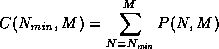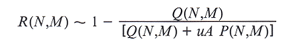VIII.D.2 Formalism for the Determination of Completeness and Reliability

# IRAS Explanatory Supplement VIII. Sky Coverage, Confusion, Completeness and Reliability D. Point Source Catalog Reliability and Completeness

## D.2 Formalism for the Determination of Completeness and Reliability

Chapter Contents | Authors | References

## D.2.a Completeness

It is necessary to estimate the fraction of real sources on the sky, above some limiting flux density value, which is actually present in the catalog. Let p be the probability that a genuine source in a given flux range fails to generate an HCON. The probability P(N,M) that a source is detected on N HCONs out of a possible M HCONs is given by:

P(N,M) = mCn(1-p)NpM-N

(VIII.D.1)

where mCn is the binomial coefficient. The completeness C(Nmin, M) of a Nmin/M survey, which requires a source to have at least Nmin HCONs out of M possible in order to be included in the catalog, is then given by:(VIII.D.2)

for a survey where two HCONs are required out of a possible two HCONs, the completeness C(2,2) is given by:

C(2,2) = (1 - p)2

(VIII.D.3)

Seventy two percent of the sky was surveyed three times and a source was accepted if it was seen at least twice. For this "two-out-of-three" strategy, the completeness C(2,3) is given by.:

C(2,3) = 3(1 - p)2 p + (1 - p)3
= (1 + 2p)(1 - p)2

(VIII.D.4)

## D.2.b Reliability

Let q be the probability, per unit area of the sky, that a false source is created on a single HCON coverage of the sky. Let A be the effective area for "weeks-confirmation" (see Sections V.D, V.H.2), i.e. the area within which a series of single HCONs must fall if they are to be considered as detections of the same source. Then qA is the probability that in a single HCON coverage a false source will be created at a given point on the sky with the possibility of being confirmed with another false source at the same position on a later HCON. The probability Q(N,M) that a false source will be created in a given area N times out of M HCONs is therefore given by

Q(N,M) = MCN(qA)N (1 - qA)M - N

(VIII.D.5)

In fact, Eq. (VIII.D.5) is only true if qA « 1 since it does not allow for the possibility that more than one false source is created within A during a single HCON. This is a necessary assumption for the IRAS processing because, by its very nature, the processing can only produce one final source per HCON from an area A. The results obtained justify the approximation.

Let u be the density of true sources in a given region of the sky. The probability of detecting a true source on N out of M HCONs in a given area of the sky is uA P(N,M) where P(N,M) is given by Eq. (VIII.D.2); it is necessary that uA « 1. Hence the total probability T(N,M) of finding a source, either false or true, on N out of M HCONs in a given area of the sky is given by:

T(N,M) ~ Q(N,M) + uA P(N,M)

(VIII.D.6)

where cross terms have been neglected. The reliability R(N,M) of an N/M source is unity minus the probability that the source is false. The probability that a given source is false is the probability of finding a false source divided by the total probability of finding any source. Therefore,(VIII.D.7)

Chapter Contents | Authors | References